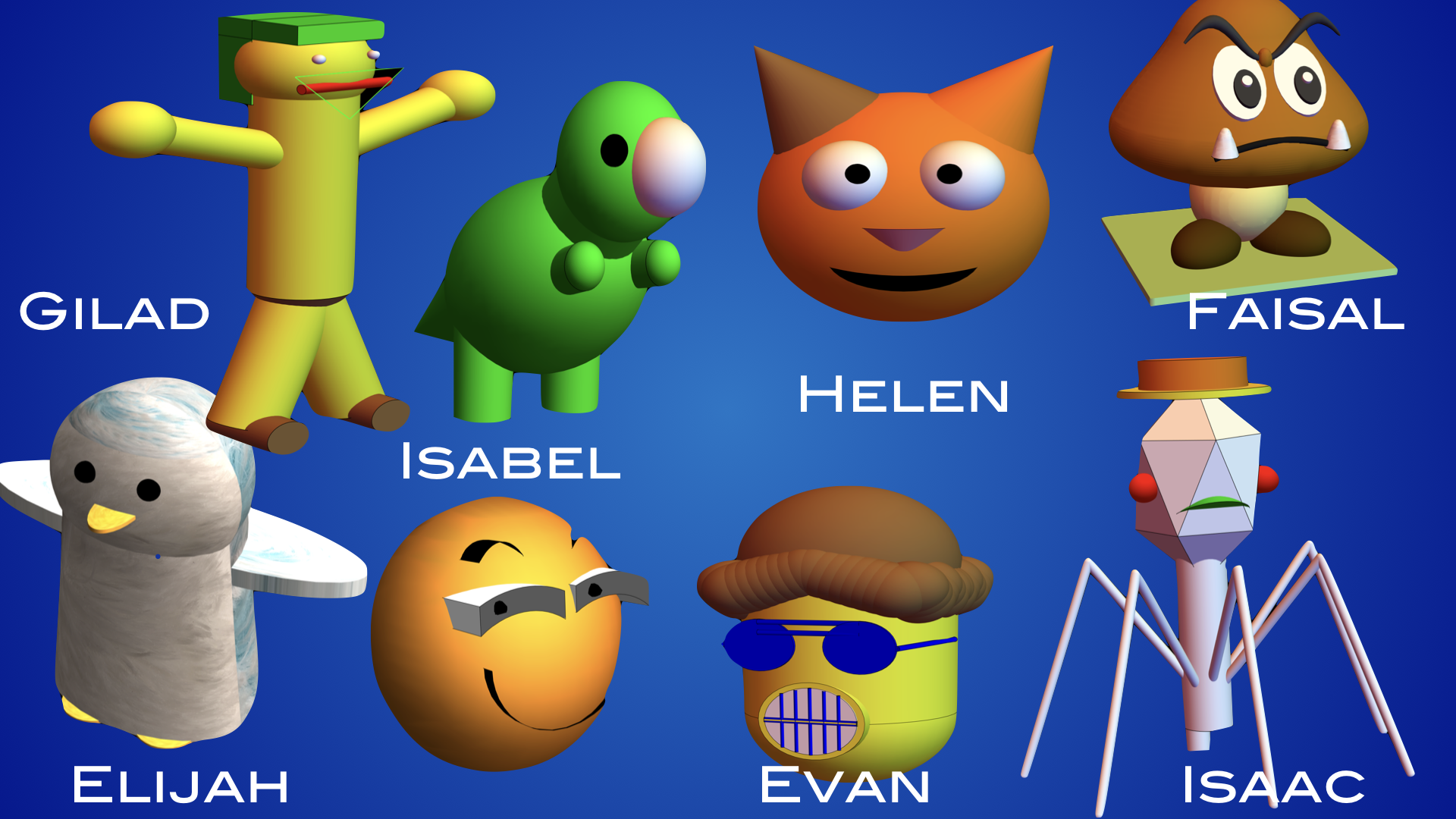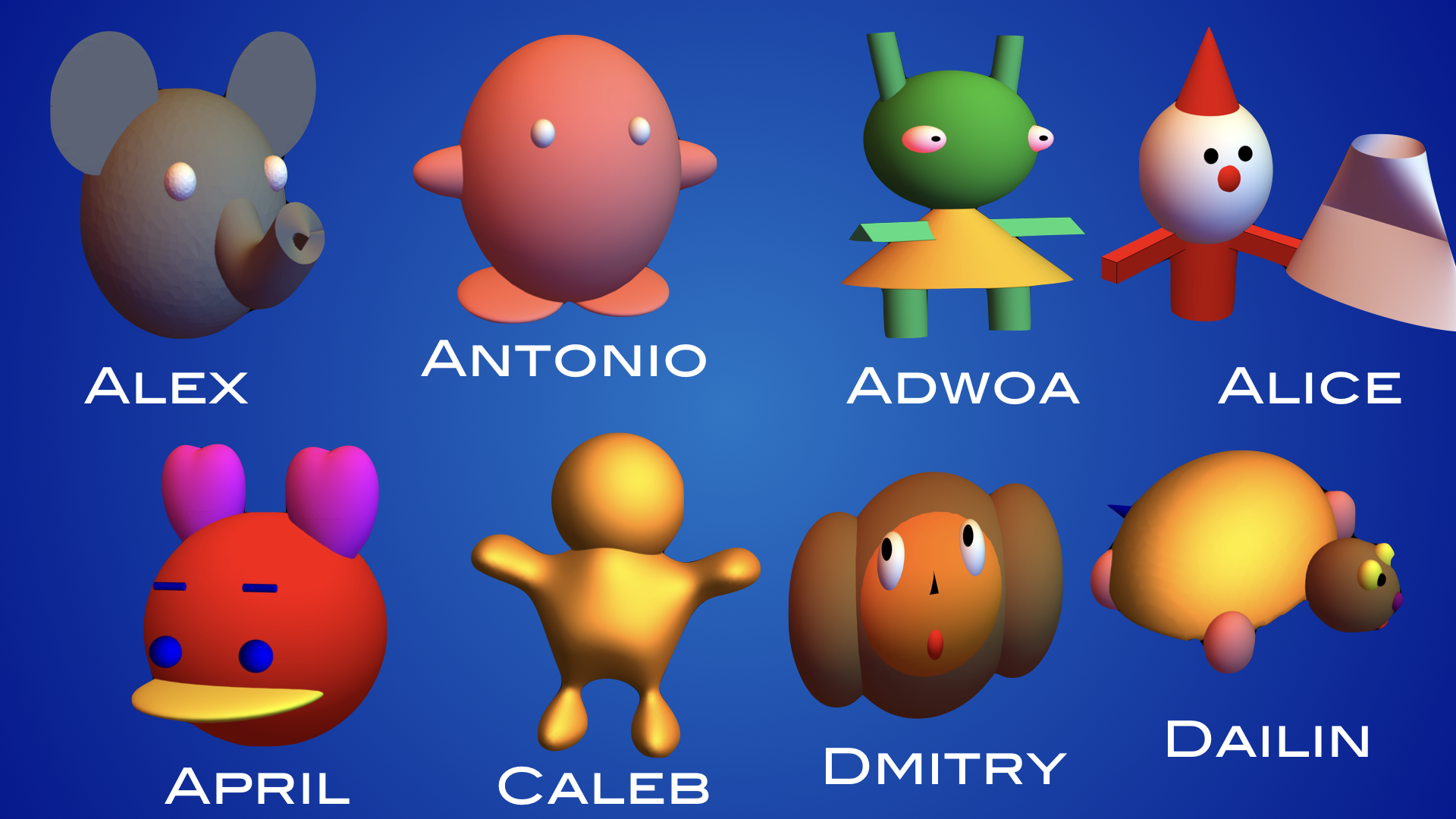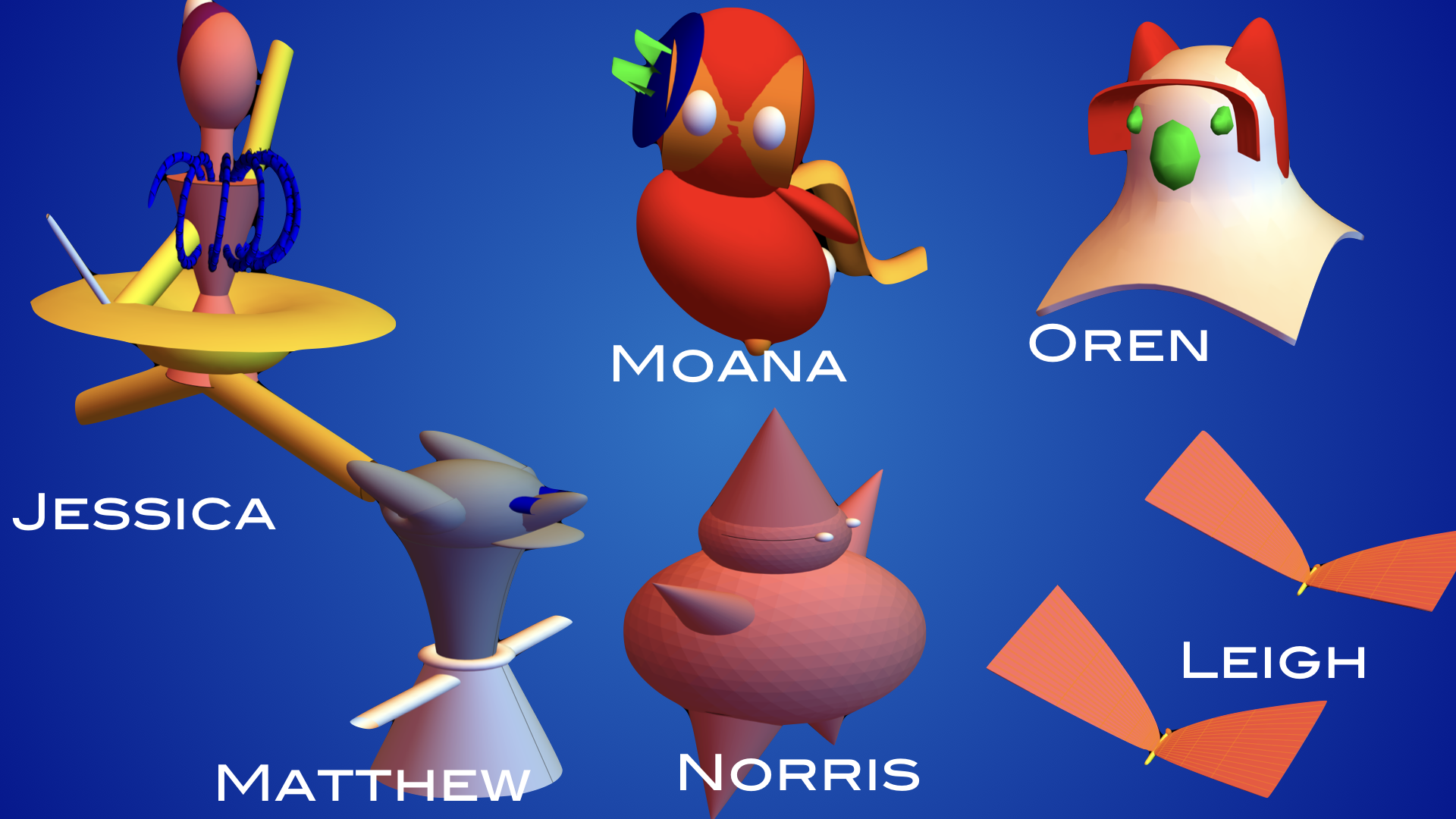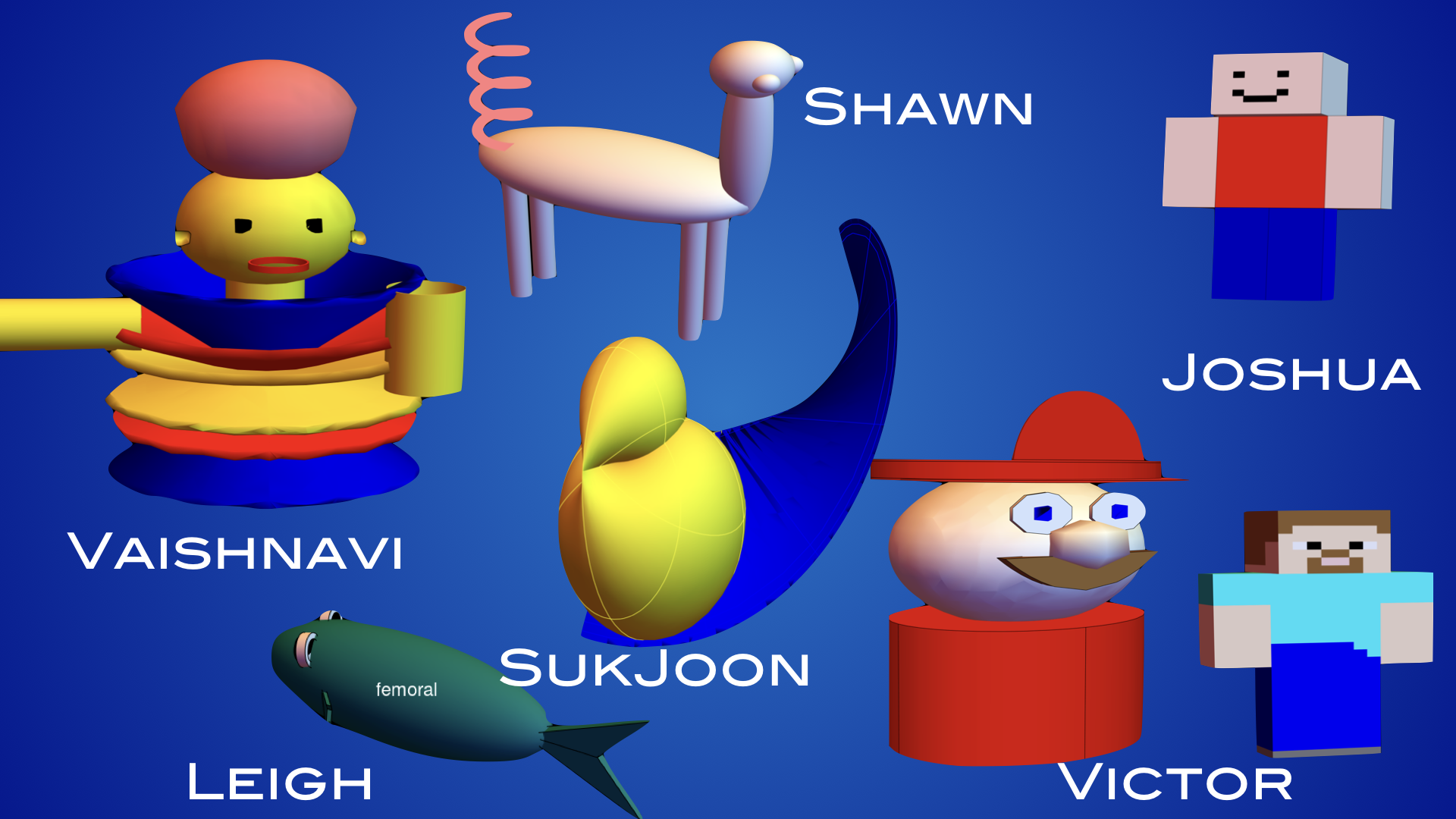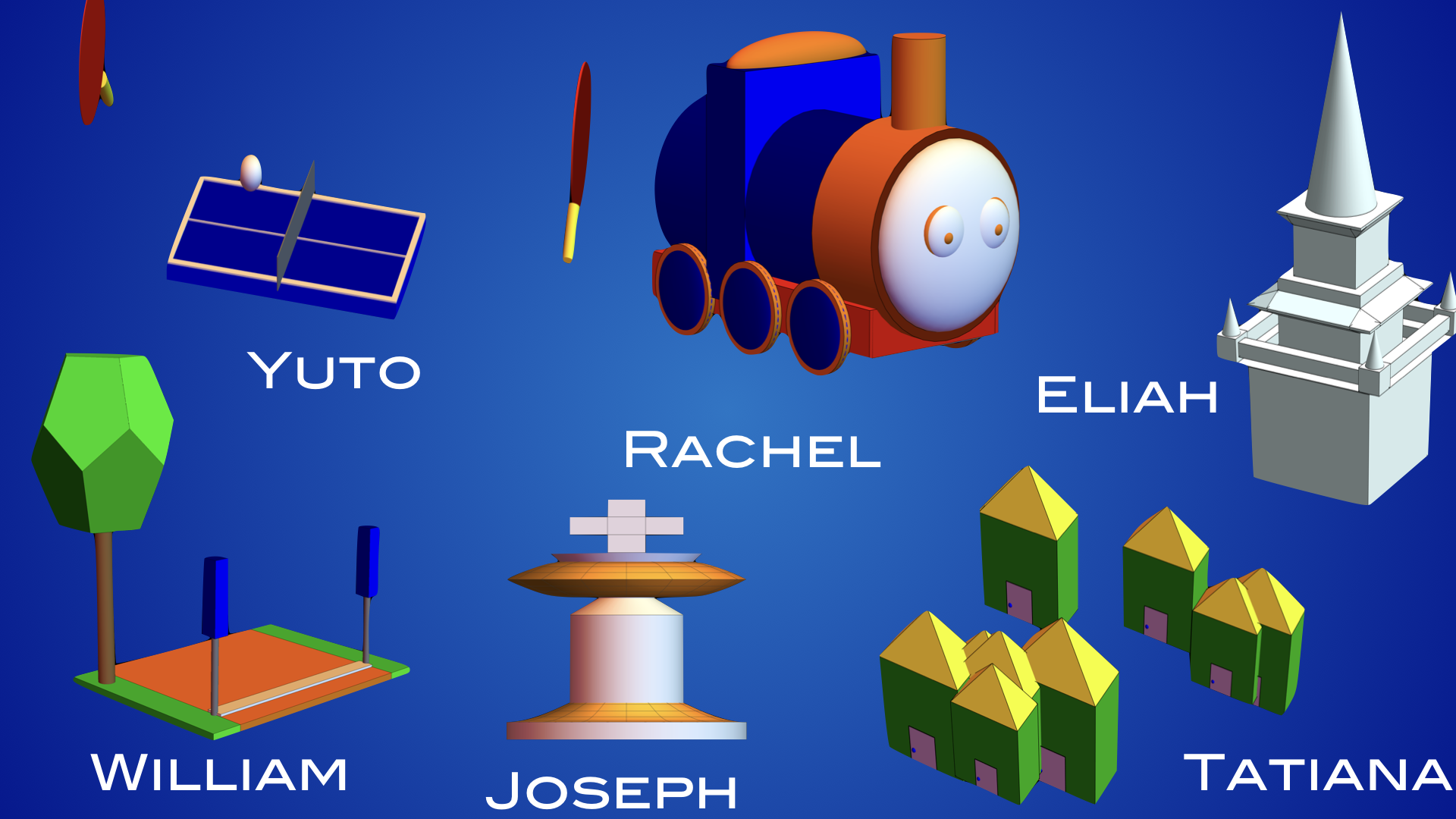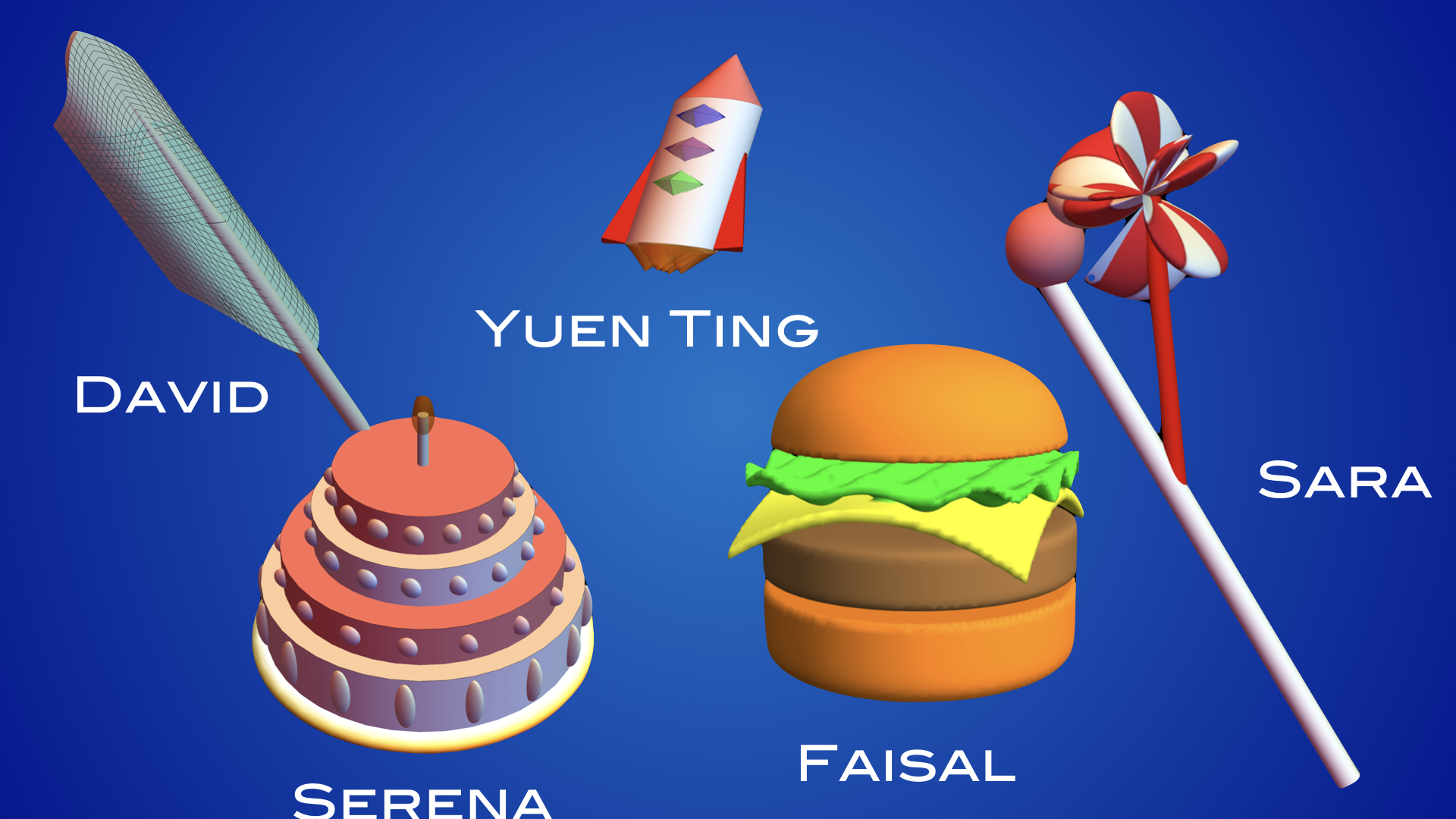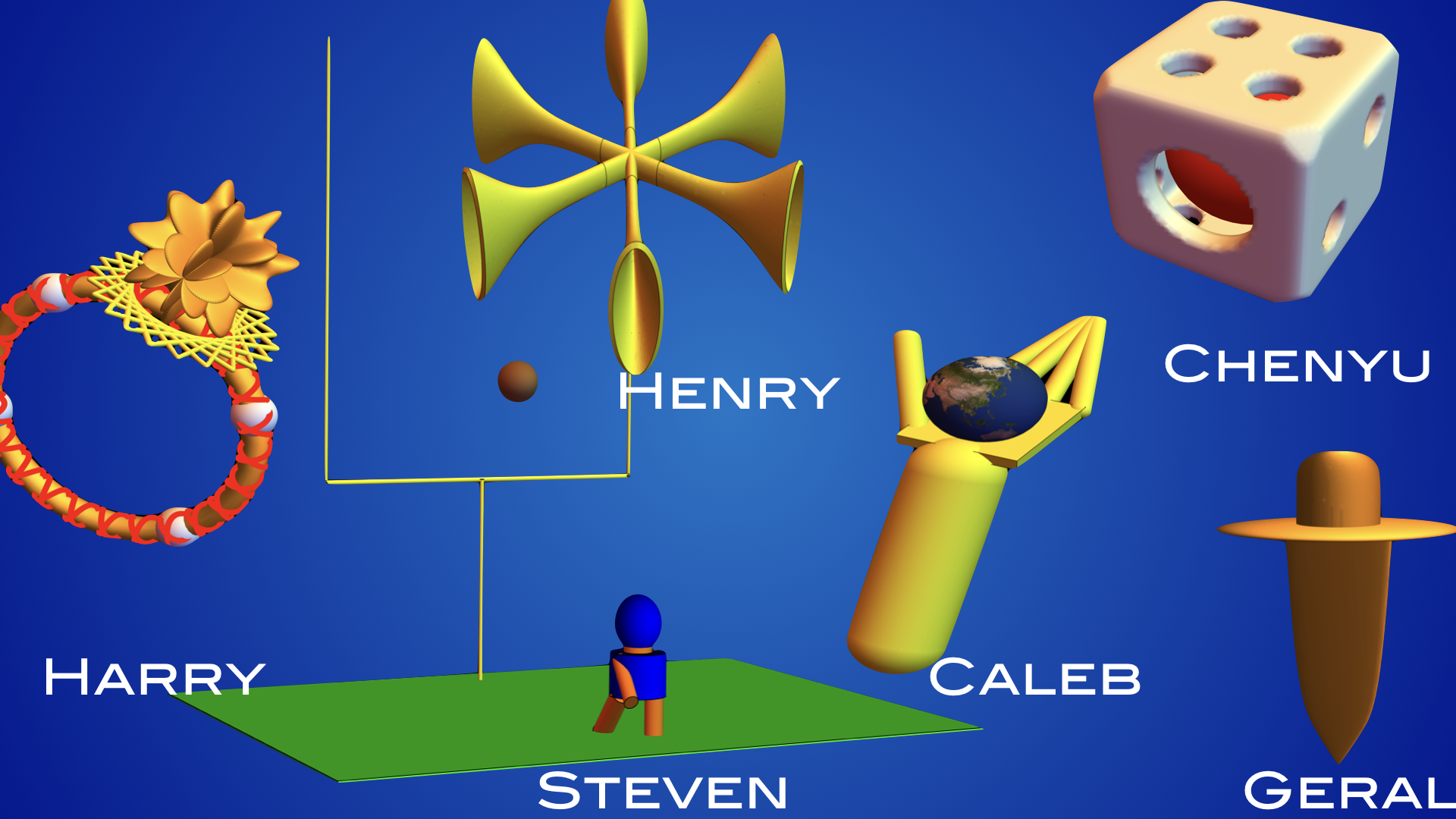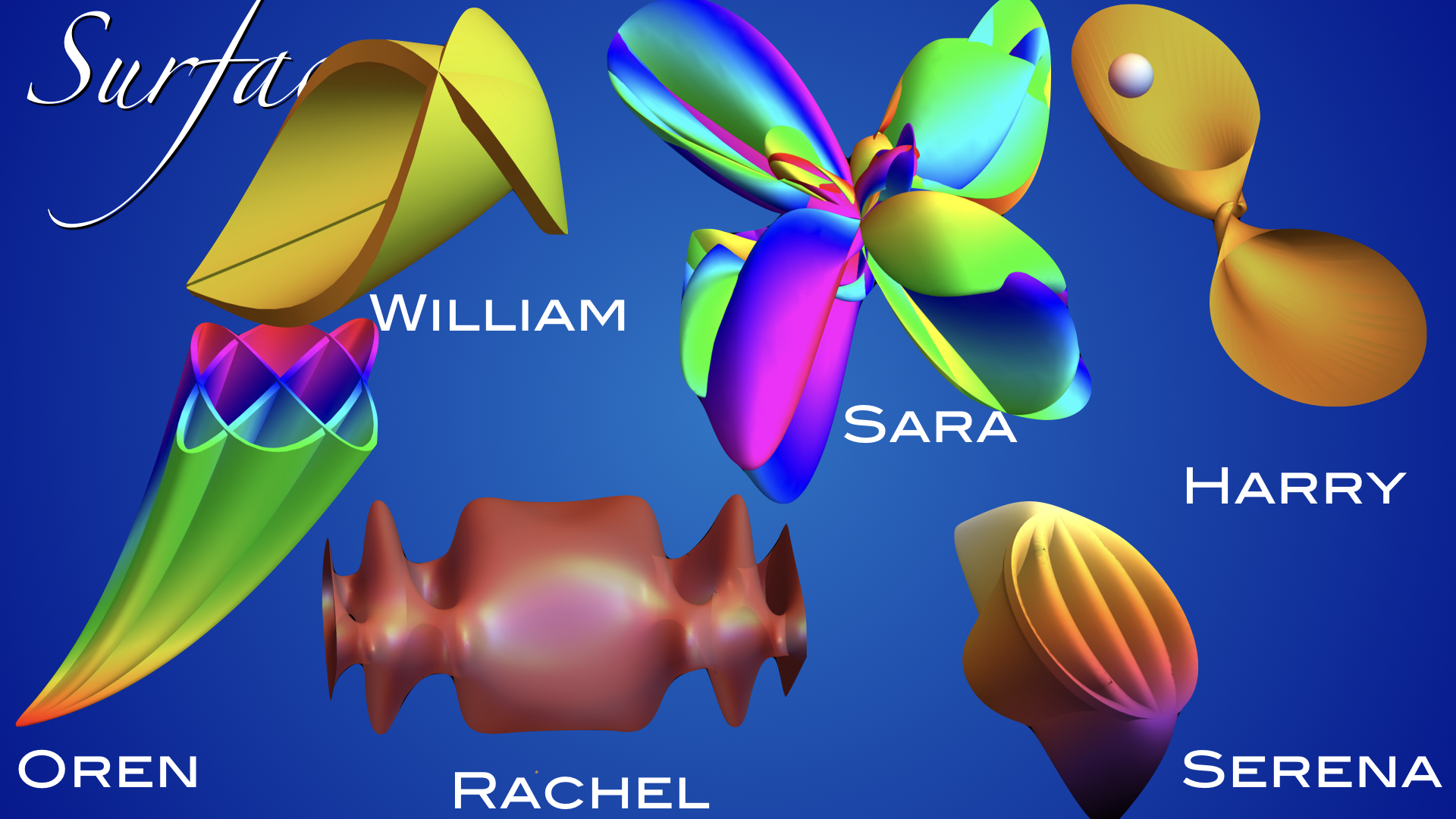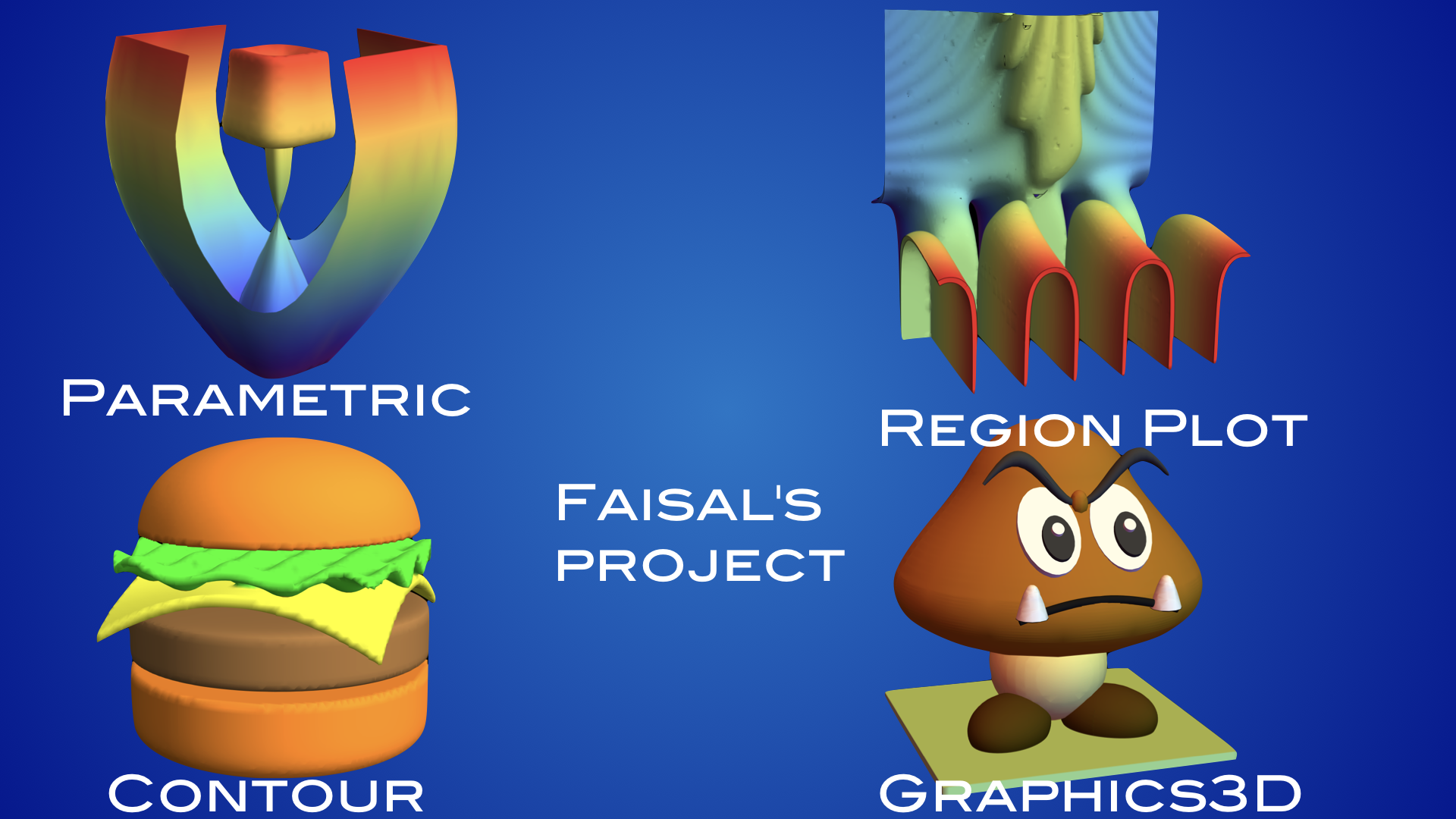# Mathematica Lab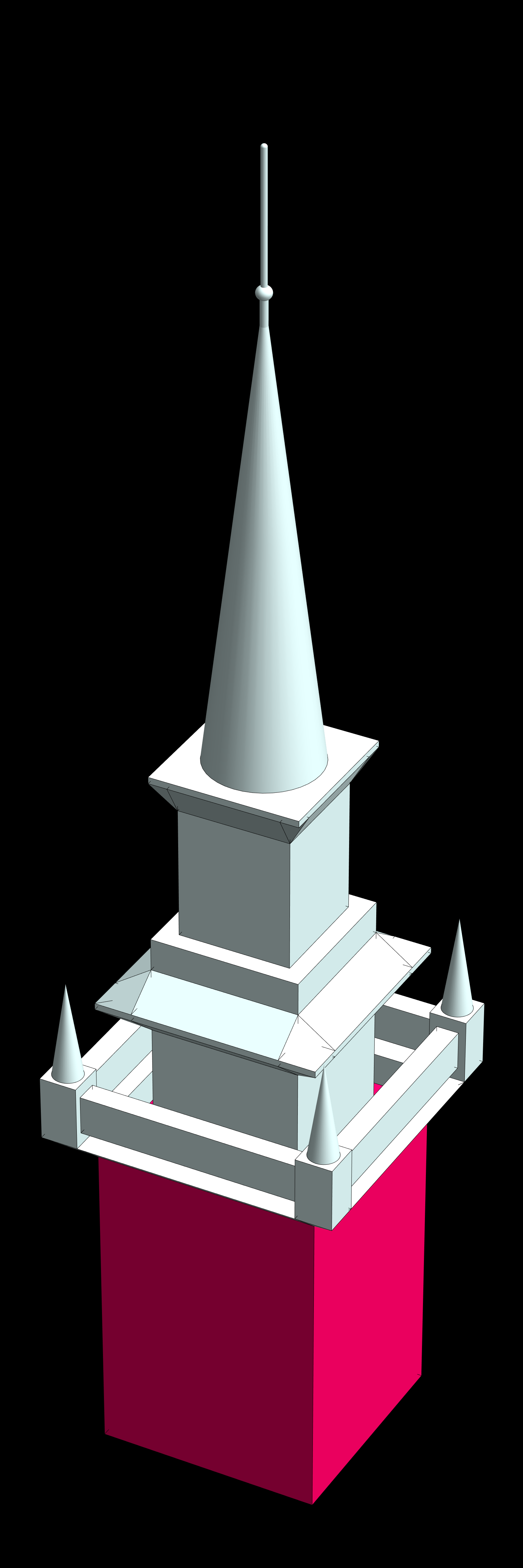Look at the exhibits page for some projects. The memorial church implementation seen to the right is due to Ziji (Elijah) Zeng. Here is the Mathematica assignment. It is due on Saturday, August 3 by email.

During the workshop, I did not emphasis enough how you can do loops and use Tubes: first tubes:
```r[t_] := {t Cos[t], t Sin[t], t};
s = Table[r[t], {t, -6 Pi, 6 Pi, 0.1}];
d = Table[2+Sin[t], {t, -6 Pi, 6 Pi, 0.1}];
S = Graphics3D[{Yellow, Tube[s,d]}]
```
Here how to produce a table of cones:
```S = Graphics3D[ Table[{Hue[Random[]/2],
Cone[{{0, 2, 0}, {Cos[t] Sin[s], Sin[t] Sin[s], Cos[s]}},0.2]},
{t, 0, 2 Pi, Pi/4}, {s, 0, Pi, Pi/4}]]
```
We used Mathematica 12 for a final project. Using the software is free for Summer school students. You will need your Harvard email address however. Start here. You have to make an account, then download and install for your platform. To download Mathematica, please go through the FAS download page.